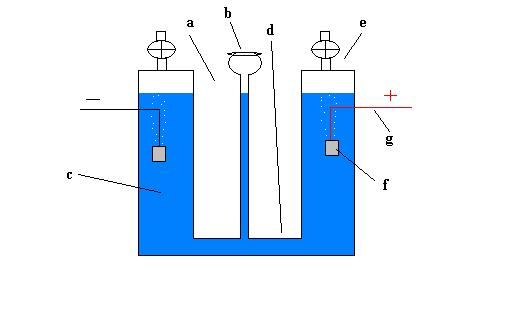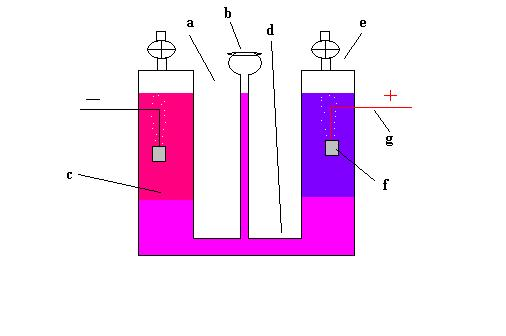The Thistle Tube Experiment

By Mike Johnston

This paper deals with my observations of a very simple experiment. One that many readers will already be familiar with from High School chemistry classes. My reason for addressing this experiment here is that it is indeed simple and easily understood but at the same time it lends itself perfectly to my goal of illustrating the fact that a container of water, with electrolyte, is not without electrical energy. It is in fact a source of electrical energy just like the power source that is used to operate the cell. I think that this concept needs to be thoroughly understood before real advances can be made in improving the efficiency of the electrolysis process. For that matter, water itself is electrically neutral but this does not imply that it is without electrical energy. A more in-depth explanation of the details of this concept are to be posted here soon but for now I want to examine this particular experiment from perhaps a slightly different perspective than is usually seen.The illustration above depicts the thistle tube experiment as it is commonly done. We see the container (a) consisting of the thistle tube (b) through which the water and electrolyte mixture (c) is added, a connecting tube (d) and two separate fluid reservoirs with petcocks at the top (e). There is one electrode in each fluid reservoir chamber (presumed to be made of a metal which is chemically inert to attack by the electrolyte being used) (f) which are connected to the leads from our DC power source (g).

When a direct current power source is connected to the leads electrolysis occurs in the unit. Hydrogen gas is produced at the cathode and Oxygen gas at the anode. The power that is supplied by the power source is said to be being converted to stored energy in the form of the released Hydrogen atoms. Efficiency is fairly good. This is the extent of the explanation on energy interactions that is usually given with this experiment.

Of course if you add a PH indicator to the solution you can see that, initially, the solution is either acid (indicator turns pink) or basic (indicator turns blue) overall, depending on the electrolyte being used. Let's say we use Sulfuric Acid as the electrolyte. The two ions present are H+ and SO4--. The solution is acidic. The indicator turns the solution pink. In each chamber there are an equal number of positive and negative ions. The SO4-- ions have a negative electrical charge of .98 volts and the H+ ions have a positive electrical charge of 0 volts. The negative is the greater charge and so the solution is electrically negative or, acid. Even though there are an equal number of both positive and negative ions and for that reason the solution can be said to be “balanced” chemically, it is not balanced or neutral electrically.After power is applied and the experiment operates for a while, we see that the solution in the cathode chamber becomes more acidic and the solution in the anode chamber becomes more basic. This is because positive H+ ions in the cathode chamber are being converted to hydrogen gas and the negative SO4-- ions are being converted to oxygen gas in the anode chamber. Because of the way that the device is constructed these ions are not able to travel to the opposite sides and react. If the experiment is allowed to run long enough electrolysis slows and eventually stops. This is due to the ongoing buildup of oppositely charged ions in each chamber.Here is where it gets interesting for me. Remember that the electrons that are picked up at the cathode are leaving the solution as hydrogen gas. This represents the energy that is being contributed to the experiment by the external power source. If this is all we consider then the experiment makes a good illustration for the conservation of energy rule and for the second law of thermodynamics. The applied energy is all accounted for (with minor resistance losses) and the world is happy.

But this isn't all that is going on here. Why, for example, does electrolysis stop after the buildup of ions as described above? There are still more ions available to react but since there is now a surplus of ions in the chamber (s) which have the same polarity as the chamber's electrode SO4-- or OH- in the cathode chamber and H+ in the anode chamber) a resistance is set up. One could call it a type of CEMF ( counter electromotive force). The electrical charge in the chambers builds up gradually as electrolysis progresses. For every electron that leaves the solution as hydrogen gas in the cathode chamber one negative ion is created in that chamber (the opposite being the case in the anode chamber). As the negative ions build up they produce a counter negative charge which eventually becomes equal to the negative charge of the cathode that is being supplied by the outside power source. This gradual buildup is why electrolysis slows and finally, when the charges in the cell are equal to the electrode charge, electrolysis stops.

At this point we have a surplus of negative ions in the cathode chamber and a surplus of positive ions in the anode chamber. Between the two types of ions there is a difference of electrical potential as I pointed out earlier. In other words we have a measurable potential difference between the two chambers. A voltage. The fact that one chamber is basic and the other acidic verifies this fact. If you disconnect the unit from the power source and connect a sensitive multimeter between the leads of the unit you may be able to measure the potential difference in this manner too.

If we could cause a current to flow between the two chambers, by connecting the leads to each other for example, how much current could flow? Current would flow until the charges in the chambers were equal. The amount of current that could flow would be exactly equal to the amount of current that flowed while the unit was under power from the outside source. Remember that current (amps) is measured in the number of electrons that flow and that for every electron that entered the unit and joined with a positive ion a negative ion with one surplus electron was created (again, the opposite is true in the anode chamber). So the amount of stored current in the chambers is then equal to the amount of current which flowed into the cell. In effect we have charged a “battery” by operating the unit as an electrolysis cell and the amount of potential energy stored in our “battery” (as oppositely charged ions) is exactly equal to the amount of energy that charged this battery.

Now consider that the energy that was delivered to the cell is already spoken for as the potential energy stored in the hydrogen atoms that were released from the cell! We now have DOUBLE the energy between the hydrogen gas and the ions in the chambers as what was originally applied. The only difference that one can point to is that the applied voltage and the voltage of either of the now existing sources of stored energy might be different. The voltage of the hydrogen is 1.23 volts when reacting with oxygen in a fuel cell and the stored energy between the chambers is .98 volts. But voltage can be manipulated so the real test is that there are surplus electrons available in both cases and positive (lacking) places for the surplus electrons to be donated to or shared with.

The only explanation for this situation is the fact that both the outside DC power source and the electrolysis cell are power sources of slightly different types. Both devices are contributing their individual energies to move electrons through the system with the hydrogen gas that is released being a by-product of the conduction process through both of the power sources. In this case both the second law of thermodynamics and the conservation of energy rule are preserved and yet the outcome is having twice the potential energy now available as was originally applied by the external power source alone.

-END-

HOME